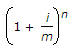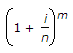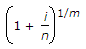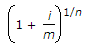# Civil Engineering - Engineering Economy

### Exercise :: Engineering Economy - Section 1

31.

If interest is paid more than once in a year, i is the rate of interest per year, n is the number of periods in years and m is a number of periods per years, compound amount factor (CAF) is :

 A.B.C.D.Explanation:

No answer description available for this question. Let us discuss.

32.

In a cash flow series :

 A. uniform gradient signifies that an income or disbursement changes by the same amount in each interest period. B. Either an increase or a decrease in the amount of a cash flow is called the gradient. C. The gradient in the cash flow may be positive or negative. D. All of these

Explanation:

No answer description available for this question. Let us discuss.

33.

If P is principal amount, i is the rate of interest and n is the number of periods in years, then the interest factor is :

 A. (1 + ni) B. (ni - 1) C. ni D. None of these

Explanation:

No answer description available for this question. Let us discuss.

34.

Pick up the correct statement from the following:

 A. Ratio analysis is the procedure of determining and interpreting numerical relationship of various items of the financial statement. B. All financial ratios are obtained by relating two sets of information contained in a Single financial statement. C. The relationship between two accounting figures expressed mathematically, is known as a financial ratio. D. All of these

Explanation:

No answer description available for this question. Let us discuss.

35.

Pick up the correct statement from the following:

 A. The ability of a company to meet obligations which are likely to mature in short term, is called liquidity. B. The liquidity ratio may be defined as a relationship of current liabilities and current assests and advances. C. The liquidity ratios are used to indicate the financial position of the firm. D. All of these